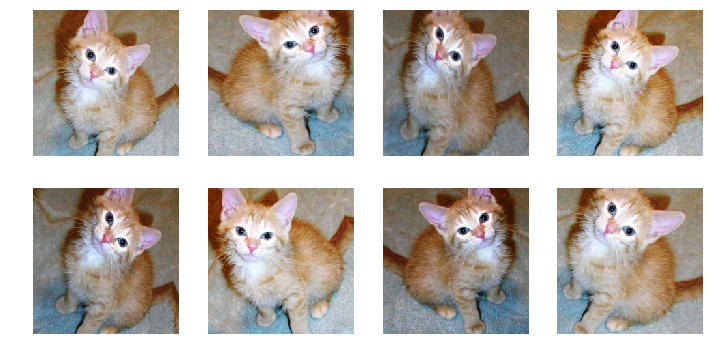List of transforms for data augmentation in CV

## Image transforms¶

fastai provides a complete image transformation library written from scratch in PyTorch. Although the main purpose of the library is data augmentation for use when training computer vision models, you can also use it for more general image transformation purposes. Before we get in to the detail of the full API, we'll look at a quick overview of the data augmentation pieces that you'll almost certainly need to use.

## Data augmentation¶

Data augmentation is perhaps the most important regularization technique when training a model for Computer Vision: instead of feeding the model with the same pictures every time, we do small random transformations (a bit of rotation, zoom, translation, etc...) that don't change what's inside the image (to the human eye) but do change its pixel values. Models trained with data augmentation will then generalize better.

To get a set of transforms with default values that work pretty well in a wide range of tasks, it's often easiest to use `get_transforms`. Depending on the nature of the images in your data, you may want to adjust a few arguments, the most important being:

• `do_flip`: if True the image is randomly flipped (default behavior)
• `flip_vert`: limit the flips to horizontal flips (when False) or to horizontal and vertical flips as well as 90-degrees rotations (when True)

`get_transforms` returns a tuple of two lists of transforms: one for the training set and one for the validation set (we don't want to modify the pictures in the validation set, so the second list of transforms is limited to resizing the pictures). This can be passed directly to define a `DataBunch` object (see below) which is then associated with a model to begin training.

Note that the defaults for `get_transforms` are generally pretty good for regular photos - although here we'll add a bit of extra rotation so it's easier to see the differences.

```tfms = get_transforms(max_rotate=25)
len(tfms)
```
`2`

We first define a function to return a new image, since transformation functions modify their inputs. We also define a little helper function `plots_f` to let us output a grid of transformed images based on a function - the details of this function aren't important here.

```def get_ex(): return open_image('imgs/cat_example.jpg')

def plots_f(rows, cols, width, height, **kwargs):
[get_ex().apply_tfms(tfms, **kwargs).show(ax=ax) for i,ax in enumerate(plt.subplots(
rows,cols,figsize=(width,height)).flatten())]
```

If we want to have a look at what these transforms actually do, we need to use the `apply_tfms` function. It will be in charge of picking the values of the random parameters and doing the transformations to the `Image` object. This function has multiple arguments you can customize (see its documentation for details); here we will highlight the most useful. The first one we'll need to set, especially if our images are of different shapes, is the target `size`. It will ensure that all the images are cropped or padded to the same size so we can then collate them into batches.

```plots_f(2, 4, 12, 6, size=224)
```Note that the target `size` can be a rectangle if you specify a tuple of int.

```plots_f(2, 4, 12, 8, size=(300,200))
```